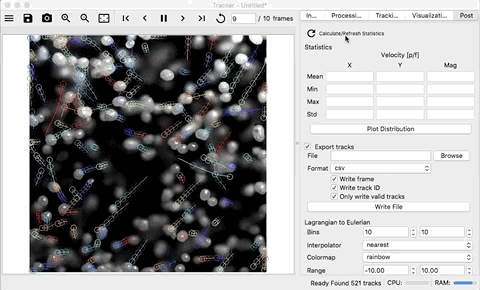# Post-Process¶

Now that objects have been tracked, statistical information can calculated. To do this, the valid tracks (stored as a dictionary) need to be converted to an array of points and velocities at those points. This is accomplished by pressing the `Calculate/Refresh Statics` button. This can take substantial time to complete. Once the process is finished, the `Mean`, Min``, `Max`, and `Std` for the `x` velocity, `y` velocity, and magnitude of velocity line-edits will be populated.

Note

Every-time the tracks change, the statistics need to be re-calculated by pressing the `Calculate/Refresh Statics` button.## Plotting Distributions¶

To see the distribution of these velocities, press the `Plot Distribution` button. This will create a new tab in the media viewer and show histograms of the velocities.

## Exporting Individual Velocities¶

The velocities can be written to a CSV file or HDF5 file (if pandas is available) be selecting the `Export tracks` check-box. Enter a path and file name in the `File` line-edit or press the browse button to select a location and file name. Use the `Write frame`, `Write track ID`, and ```Only write valid tracks``` check-boxes to include/exclude the writing of the frame the velocity was measured on, the unique track identifier, and whether or not to write only the valid tracks or all the tracks, respectively. To actually write the file, press the `Write File` button.

When running without the GUI, if the `Export tracks` check-box is selected and a valid path is provided, the file will be written after all the specified frames have been processed.

A 2D array will be written where the rows are individual velocity measurements and the columns will depend on what is selected:

```[frame,] [track id,] x position, y position, x velocity, y velocity, magnitude
```

The HDF5 file will have an array named tracks which is the 2D array describe above. This file can be read using pandas:

```# import pandas
import pandas as pd

# print the columns
print(df.columns)
# Index(['frame', 'track_id', 'x_pos', 'y_pos', 'x_vel', 'y_vel', 'mag'], dtype='object')

# the columns can be indexed using the name
df['x_vel']
```

Note

The HDF5 file will be much smaller than the ASCII CSV file as well as faster to read.

## Lagrangian to Eulerian¶

To help reduce and interpret the individual velocity measurements, the lagrangian data can be binned spatially by position. Statistics can then be calculated for the x and y velocities that fall into a particular bin. The mean velocities can by plotted by pressing the `Plot Grid` button and the resulting vector, colored and scaled by the magnitude can be plotted by pressing the `Plot Quiver` button.

The number of bins in the x and y directions can be changed using the `Bins` spinneres. The interpolation technique for the grid plots can be changed using the `Interpolator` drop-down list. The color map for both the grid plots and the quiver plot can be changed using the `Colormap` drop-down list. The range used for the color map can be specified using the `Range` spinneres.

## Export Eulerian Data¶

The Eulerian data can be saved by selecting the `Export Eulerian Grid` check-box. Enter a path and file name in the `File` line-edit or press the browse button to select a location and file name. Select a file format from the `Format` drop-down list. To actually write the file, press the `Write File` button. The X velocity, Y velocity, and magnitude will all be determined and written.

When running without the GUI, if the `Export Eulerian Grid` check-box is selected and a valid path is provided, the file will be written after all the specified frames have been processed.

### HDF File¶

The HDF file will have all three arrays saved inside as datasets. This file can be read back in using the h5py library:

```import h5py

f = h5py.File('path/to/file/hdf', 'r')

# print the dataset keys
print(list(f.keys()))

# the datasets indexed using the name
f['x_vel']

# the array can be plotted with matplotlib
from matplotlib import pyplot as plt
plt.imshow(f['x_vel'])
plt.show()
```

### Numpy File¶

The numpy files can not handle multiple arrays in the same file. As a results, each array (`x_vel`, `y_vel`, `mag_vel`, `x`, `y`) is saved as a different file.

### CSV File¶

The csv files can not handle multiple arrays in the same file. As a results, each array (`x_vel`, `y_vel`, `mag_vel`, `x`, `y`) is saved as a different file.